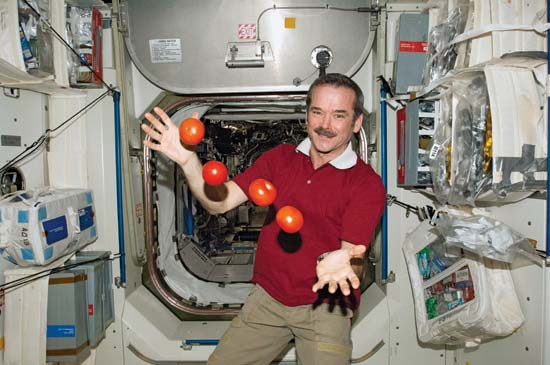# WeightlessWhat is the period of rotation (in minutes) of the Earth so as to make any object weight-less on its equator?

Details and Assumptions

• The radius of the Earth is $R = 6 \times 10^{6}\text{ m}.$
• The gravitational acceleration is $g = 9.8\text{ m/s}^2.$
×

Problem Loading...

Note Loading...

Set Loading...